Firing & Commutation Circuits of SCR

# Firing & Commutation Circuits of SCR - Power Electronics - Electrical Engineering (EE)

Two Transistor analogy of SCR

The principle of thyristor operation can be explained with the use of its two-transistor model (or two-transistor analogy). Fig. 4.15 (a) shows schematic diagram of a thyristor. From this figure, two-transistor model is obtained by bisecting the two middle layers, along the dotted line, in two separate halves as shown in Fig. 4.15 (b). In this figure, junctions J1 – j2and J2 -J3 can be considered to constitute pnp and npn transistors separately. The circuit representation of the two-transistor model of a thyristor is shown in Fig. 4.15 (c).
In the off-state of a transistor, collector current Ic is related to emitter current IE as
IC = αIE + ICBO
where α is the common-base current gain and ICB0 is the common-base leakage current of collector-base junction of a transistor.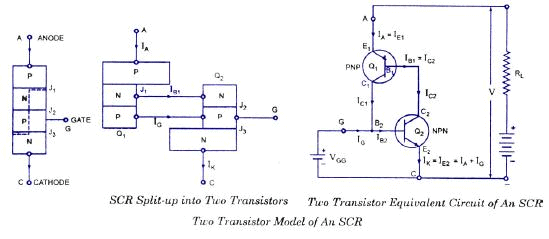For transistor Q1 in Fig. 4.15 (c), emitter current I= anode current Ia and IC = collector current IC1. Therefore, for Q1
IC1 = αIa + ICBO1 ……..(4.3)
where α1 = common-base current gain of Q1
and ICBO1 = common-base leakage current of Q1
Similarly, for transistor Q2, the collector current IC2 is given by
IC2 = α2 Ik + ICBO2 …(4.4)

where α2 – common-base current gain of Q2,ICBO2 =common-base leakage current of Q2 and
Ik = emitter current of Q2.
The sum of two collector currents given by Eqs. (4.3) and (4.4) is equal to the external circuit current Iα entering at anode terminal A.
There fore Ia = IC1 + IC2
Ia = α1 Ia + ICBO1+ α2 Ik + ICBO2 …(4.5)
When gate current is applied, then Ik = Ia + Ig . Substituting this value of Ik in Eq. (4.5) gives
Ia = α1 Ia + ICBO1+ α2 (Ia + Ig ) + ICBO2
or
Ia = α2 Ig + ICBO1 + ICBO2 /[1-( α1+ α2)]

For a silicon transistor, current gain α is very low at low emitter current. With an increase in emitter current, a builds up rapidly as shown in Fig. 4.16. With gate current Ig = 0 and with thyristor forward biased,( α1+ α2)is very low as per Eq (4.6) and forward leakage current somewhat more than ICBO1 + ICBO2 flows. If, by some means, the emitter current of two component transistors can be increased so that α1+ α2 approaches unity, then as per Eq. (4.6) Ia would tend to become infinity thereby turning-on the device. Actually, external load limits the anode current to a safe value after the thyristor begins conduction. The methods of turning-on a thyristor, in fact, are the methods of making α1+ α2 to approach unity. These 0.25 various mechanisms for turning-on a thyristor are now discussed below :

(i) GATE Triggering : With anode positive with respect to cathode and with gate current Ig = 0, Eq. (4.6) shows that anode current, equal to the forward leakage current, is somewhat more than ICBO1 + ICBO2,Under these conditions, the device is in the forward blocking state.
Now a sufficient gate-drive current between gate and cathode of the transistor is applied. This gate-drive current is equal to base current IB2 = Ig and emitter current Ik of transistor Q2. With the establishment of emitter current Ik of Q2, current gain α2 of Q2 increases and base current IB2 causes the existence of collector current IC2 = β2IB2 = β2 Ig. This amplified current IC2 serves as the base current IB1 of transistor Q1 With the flow of IB1 collector current IC1 = β1 IB1 = β1 β2 Ig of Q1comes into existence. Currents IB1 and IC1 lead to the establishment of emitter current Ia of Q1 and this causes current gain α1 to rise as desired. Now current Ig + ICI = (1 + β1 β2) Ig acts as the base current of Q2 and therefore its emitter current Ik = ICI + Ig With the rise in emitter current Ik α2 of Q2 increases and this further causes IC2 = P2 (1 + β1 β2) Ig to rise. As amplified collector current IC2 is equal to the base current of Q1 current gain α1 eventually rises further. There is thus established a regenerative action internal to the device. This regenerative or positive feedback effect causes α1+ αto grow towards unity. As a consequence, anode current begins to grow towards a larger value limited only by load impedance external to the device. When regeneration has grown sufficiently, gate current can be withdrawn. Even after Igis removed, regeneration continues. This characteristic of the thyristor makes it suitable for pulse triggering. Note that thyristor is a latching device

After thyristor is turned on, all the four layers are filled with carriers and all junctions are forward biased. Under these conditions, thyristor has very low impedance and is in the forward on-state.

(ii) Forward-voltage triggering : If the forward anode to cathode voltage is increased, the collector to emitter voltages of both the transistors are also increased. As a result, the leakage current at the middle junction J2 of thyristor increases, which is also the collector current of Q2 as well as Q1 With increase in collector currents IC1 and IC2 due to avalanche effect, the emitter currents of the two transistors also increase causing α1+ α2 to approach unity. This leads to switching action of the device due to regenerative action. The forward-voltage triggering for turning-on a thyristor may be destructive and should therefore be avoided.

(iii) dv/dt triggering : The reversed biased junction J2 behaves like a capacitor because of the space-charge present there. Let the capacitance of this junction be Cj. For any capacitor, i = C dv/dt.In case it is assumed that entire forward voltage va appears across reverse biased junction J2 then charging current across the junction is given by
i = Cj dva /dt
This charging or displacement current across junction J2 is collector currents of Q2 and Q1 Currents IC2, IC1 will induce emitter current in Q2, Q1 In case rate of rise of anode voltage is large, the emitter currents will be large and as a result, α1+ αwill approach unity leading to eventual switching action of the thyristor.

(iv) Temperature triggering : At high temperature, the forward leakage current across junction J2 rises. This leakage current serves as the collector junction current of the component transistors Q1 and Q2. Therefore, an increase in leakage current ICI, IC2 leads to an increase in the emitter currents of QQ2. As a result, (α1+ α2) approaches unity. Consequently, switching action of thyristor takes place.

(v) Light triggering : When light is thrown on silicon, the electron-hole pairs increase. In the forward-biased thyristor, leakage current across J2 increases which eventually increases α1+ α2 to unity as explained before and switching action of thyristor occurs.
As stated before, gate-triggering is the most common method for turning-on a thyristor. Light-triggered thyristors are used in HVDC applications.
The operational differences between thyristor-family and transistor family of devices may now be summarised as under :

i) Once a thyristor is turned on by a gate signal, it remains latched in on-state due to internal regenerative action. However, a transistor must be given a continuous base signal to remain in on-state.

ii) In order to turn-off a thyristor, a reverse voltage must be applied across its anode-cathode terminals. However, a transistor turns off when its base signal is removed.

2.2 Different Firing Circuits of SCR: One common application of the uni junction transistor is the triggering of the other devices such as the SCR, triac etc. The basic elements of such a triggering circuit are shown in figure. The resistor RE is chosen so that the load line determined by RE passes through the device characteristic in the negative resistance region, that is, to the right of the peak point but to the left of the valley point, as shown in figure. If the load line does not pass to the right of the peak point P, the device cannot turn on. For ensuring turn-on of UJT
RE < VBB – Vp / I
This can be established as below
Consider the peak point at which IRE = Ip and VE = VP (the equality IRE = IP is valid because the charging current of capacitor, at this instant is zero, that is, the capacitor, at this particular instant, is changing from a charging state to a discharging state). Then VE = VBB – IRE R
So, RE(MAX) = VBB – VE / IRE = VBB – Vp / IP at the peak point. At the valley point, V IE = IV and VE = VV so that
VE = VBB – IRE R
So RE(MIN) = VBB – VE / IRE = VBB – V/ IV or for ensuring turn-off.
RE > = VBB – VV / IV
So, the range of resistor RE is given as
VBB – VP / IP >RE > VBB – VV / IV
The resistor R is chosen small enough so as to ensure that SCR is not turned on by voltage VR when emitter terminal E is open or IE = 0
The voltage VR = RVBB/R + RBB for open-emitter terminal.
The capacitor C determines the time interval between triggering pulses and the time duration of each pulse. By varying RE, we can change the time constant RE C and alter the point at which the UJT fires. This allows us to control the conduction angle of the SCR, which means the control of load current.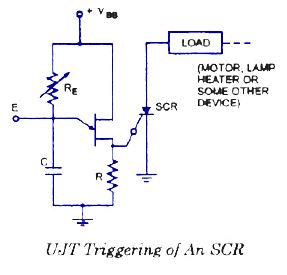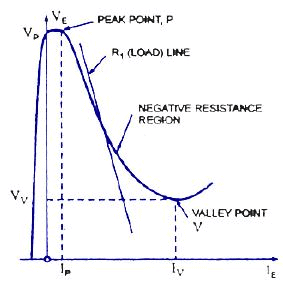Series and Parallel connections of SCRs

In many power control applications the required voltage and current ratings exceed the voltage and current that can be provided by a single SCR. Under such situations the SCRs are required to be connected in series or in parallel to meet the requirements. Sometimes even if the required rating is available, multiple connections are employed for reasons of economy and easy availability of SCRs of lower ratings. Like any other electrical equipment, characteristics/properties of two SCRs of same make and ratings are never same and this leads to certain problems in the circuit. The mismatching of

SCRs is due to differences in

(i) turn-on time
(ii) turn-off time
(iii) leakage current in forward direction
(iv) leakage current in reverse direction and
(v) recovery voltage.

Series Connection of an SCR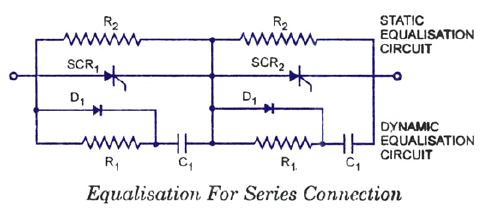When the required voltage rating exceeds the SCR voltage rating, a number of SCRs are required to be connected in series to share the forward and reverse voltage. As it is not possible to have SCRs of completely identical characteristics, deviation in characteristics lead to the following two major problems during series connections of the SCRs:

(i) Unequal distribution of voltage across SCRs.

(ii) Difference in recovery characteristics.

Care must be taken to share the voltage equally. For steady-state conditions, voltage sharing is achieved by using a resistance or a Zener diode in parallel with each SCR. For transient voltage sharing a low non-inductive resistor and capacitor in series are placed across each SCR, as shown in figure. Diodes D1 connected in parallel with resistor Rl, helps in dynamic stabilisation. This circuit reduces differences between blocking voltages of the two devices within permissible limits. Additionally the R-C circuit can also serve the function of ‘snubber circuit‘. Values of R1 and C1 can primarily be calculated for snubber circuit and a check can be made for equali-zation. If ΔQ is the difference in recovery charge of two devices arising out of different recovery current for different time and ΔV is the permissible difference in blocking voltage then C= ΔQ/ ΔV.

The value of resistance Rx should be sufficient to over damp the circuit. Since the capacitor C1 can discharge through the SCR during turn-on, there can be excessive power dissipation, but the switching current from C1 is limited by the resistor R1 This resistance also serves the purpose of damping out ‘ringing’ which is oscillation of C1 with the circuit inductance during commutation. All the SCRs connected in series should be turned-on at the same time when signals are applied to their gates simultaneously.

Parallel Connection of an SCR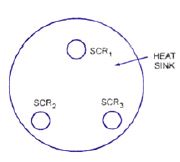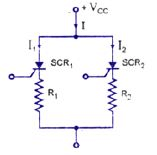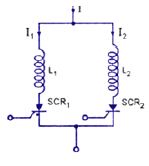When the load current exceeds the SCR current rating, SCRs are connected in parallel to share the load current. But when SCRs are operated in parallel, the current sharing between them may not be proper. The device having lower dynamic resistance will tend to share more current. This will raise the temperature of that particular device in comparison to other, thereby reducing further its dynamic resistance and increasing current through it. This process is cumulative and continues till the device gets punctured.

Some other factors which directly or indirectly add to this problem are difference in turn-on time, delay time, finger voltage* and loop inductance. Arrangement of SCRs in the cubicle also plays vital role. When the SCRs are connected in parallel, it must be ensured that the latching current level of the all the SCRs is such that when gate pulse is applied, all of them turn-on and remain on when the gate pulse is removed. Further the holding currents of the devices should not be so much different that at reduced load current one of the device gets turned-off because of fall of current through it blow its holding current value. This is particularly important because on increase in load current, the device which has stopped conducting cannot start in the absence of gate pulse.

Another point to be considered is the on-state voltage across the device. For equal sharing of currents by the devices voltage drop across the parallel paths must be equal. For operation of all the SCRs connected in parallel at the same temperature, it becomes necessary to use a common heat sink for their mounting, as illustrated in figure. Resistance compensation used for dc circuits is shown in figure. In this circuit the resistors Rand R2 are chosen so as to cause equal voltage drop in both arms. Inductive compensation used for ac circuits is shown in figure The difference in characteristics due to different turn-on time, delay time, finger voltage, latching current,holding current can be minimized by using inductive compensation. Firing circuits giving high rate of rise can be used to reduce mismatch of gate characteristics and delay time. Current sharing circuits must be designed so as to distribute current equally at maximum temperature and maximum anode current. This is done to ensure that the devices share current equally under worst operating conditions. Mechanical arrangement of SCRs also plays an important role in reducing mismatching. Cylindrical construction is perhaps the best from this point of view.

Derating. Even with all the measures taken, it is preferable to derate the device for series/parallel operation. Another reason for derating is poor cooling and heat dissipation as number of devices operate in the same branch of the circuit. Normal derating factors are 10 to 15% for parallel connection of SCRs depending upon the number of devices connected in parallel. Higher voltage safety factor is taken when SCRs are connected in series.

Commutation circuits

• Requirements to be satisfied for the successful turn-off of a SCR
• The turn-off groups as per the General Electric classification
• The operation of the turn-off circuits
• Design of a SCR commutation circuit

A thyristor can be turned ON by applying a positive voltage of about a volt or a current of a few tens of milliamps at the gate-cathode terminals. However, the amplifying gain of this regenerative device being in the order of the 108, the SCR cannot be turned OFF via the gate terminal. It will turn-off only after the anode current is annulled either naturally or using forced commutation techniques. These methods of turn-off do not refer to those cases where the anode current is gradually reduced below Holding Current level manually or through a slow process. Once the SCR is turned ON, it remains ON even after removal of the gate signal, as long as a minimum current, the Holding Current, Ih, is maintained in the main or rectifier circuit.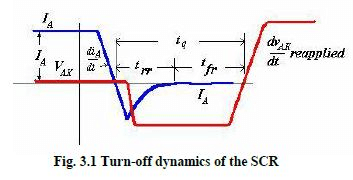In all practical cases, a negative current flows through the device. This current returns to zero only after the reverse recovery time trr, when the SCR is said to have regained its reverse blocking capability. The device can block a forward voltage only after a further tfr, the forward recovery time has elapsed. Consequently, the SCR must continue to be reverse-biased for a minimum of tfr + trr = tq, the rated turn- off time of the device. The external circuit must therefore reverse bias the SCR for a time toff > tq. Subsequently, the reapplied forward biasing voltage must rise at a dv/dt < dv/dt (reapplied) rated. This dv/dt is less than the static counterpart. General Electric has suggested six classification methods for the turn-off techniques generally adopted for the SCR. Others have chosen different classification rules.
SCRs have turn-off times rated between 8 - 50 μsecs. The faster ones are popularly known as 'Inverter grade' and the slower ones as 'Converter grade' SCRs. The latter are available at higher current levels while the faster ones are expectedly costlier.

Classification of forced commutation methods

The six distinct classes by which the SCR can be turned off are:

Class A              Self commutated by a resonating load
Class B              Self commutated by an L-C circuit
Class C             C or L-C switched by another load carrying SCR
Class D             C or L-C switched by an auxiliary SCR

Class E An external pulse source for commutation Class
F AC line commutation
These examples show the classes as choppers. The commutation classes may be used in practice in configurations other than choppers.

Class A, Self commutated by resonating the load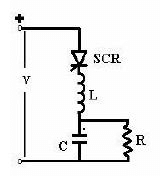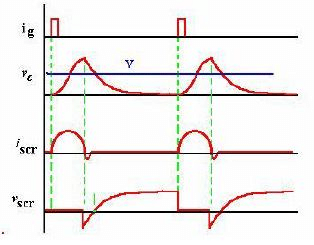Fig. 3.2 A resonant load commutated SCR and the corresponding waveforms

When the SCR is triggered, anode current flows and charges up C with the dot as positive. The L-C-R form a second order under-damped circuit. The current through the SCR builds up and completes a half cycle. The inductor current will then attempt to flow through the SCR in the reverse direction and the SCR will be turned off.
The capacitor voltage is at its peak when the SCR turns off and the capacitor discharges into the resistance in an exponential manner. The SCR is reverse-biased till the capacitor voltages returns to the level of the supply voltage V.

Class B, Self commutated by an L-C circuit

The Capacitor C charges up in the dot as positive before a gate pulse is applied to the SCR. When SCR is triggered, the resulting current has two components.
The constant load current Iload flows through R - L load. This is ensured by the large reactance in series with the load and the freewheeling diode clamping it. A sinusoidal current flows through the resonant L- C circuit to charge-up C with the dot as negative at the end of the half cycle. This current will then reverse and flow through the SCR in opposition to the load current for a small fraction of the negative swing till the total current through the SCR becomes zero. The SCR will turn off when the resonant–circuit (reverse) current is just greater than the load current.
The SCR is turned off if the SCR remains reversed biased for tq > toff, and the rate of rise of the reapplied voltage < the rated value.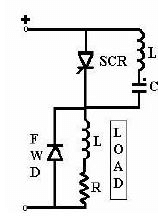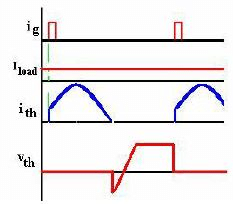Problem #1
A Class B turn-off circuit commutates an SCR. The load current is constant at 10 Amps. Dimension the commutating components L and C. The supply voltage is 100VDC.

Soln # 1
The commutating capacitor is charged to the supply voltage = 100 V
The peak resonant current is,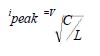Assuming,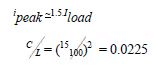The SCR commutates when the total current through it reaches zero.This corresponds to 0.73 rads after the zero crossing of the resonant current. The capacitor voltage at that instant is 75 volts. After the SCR turns off, the capacitor is charged linearly by the load current.

If the SCR is to commutate at twice this load current, for a rated "Inverter grade' SCR turn-off time of 20 μsecs,

It can be observed that if the peak of the commutating current is just equal to the load current, the turn-off time would be zero as the capacitor would not be able to impress any negative voltage on the SCR.

Class C, C or L-C switched by another load–carrying SCR

This configuration has two SCRs. One of them may be the main SCR and the other auxiliary. Both may be load current carrying main SCRs. The configuration may have four SCRs with the load across the capacitor, with the integral converter supplied from a current source. Assume SCR2 is conducting. C then charges up in the polarity shown. When SCR1 is triggered, C is switched across SCR2 via SCR1 and the discharge current of C opposes the flow of load current in SCR2.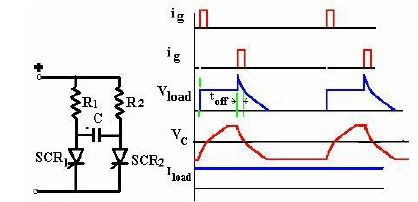Fig. 3.4 Class C turn-off, SCR switched off by another load-carring SCR

Class D, L-C or C switched by an auxiliary SCR

Example 1

The circuit shown in Figure 3.3 (Class C) can be converted to Class D if the load current is carried by only one of the SCR’s, the other acting as an auxiliary turn-off SCR. The auxiliary SCR would have a resistor in its anode lead of say ten times the load resistance.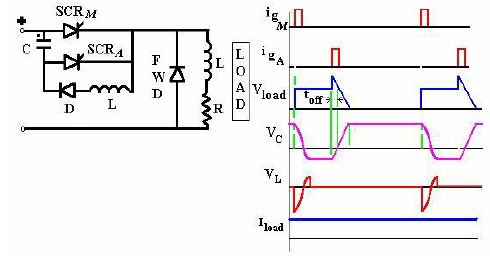Fig. 3.5 Class D turn-off. Class D commutation by a C (or LC) switched by an Auxiliary SCR.

Example 2

SCRA must be triggered first in order to charge the upper terminal of the capacitor as positive. As soon as C is charged to the supply voltage, SCRA will turn off. If there is substantial inductance in the input lines, the capacitor may charge to voltages in excess of the supply voltage. This extra voltage would discharge through the diode-inductor-load circuit.
When SCRM is triggered the current flows in two paths: Load current flows through the load and the commutating current flows through C- SCRM -L-D network. The charge on C is reversed and held at that level by the diode D. When SCRA is re-triggered, the voltage across C appears across SCRM via SCRA and SCRM is turned off. If the load carries a constant current as in Fig. 3.4, the capacitor again charges linearly to the dot as positive.

Problem # 2

A Class D turn-off circuit has a commutating capacitor of 10 μF. The load consists of a clamped inductive load such that the load current is reasonably constant at 25 amperes. The 'Inverter grade' SCR has a turn-off time of 12 μsecs. Determine whether the SCR will be satisfactorily commutated. Also dimension the commutating inductor. The supply voltage is 220 VDC.

Soln # 2

The capacitor is initially charged to the supply voltage 220 V at the end of the conduction period of SCRA.
When SCRM is triggered, the 25 Amps load current and the L-C ringing current flows through it. Peak current through SCR is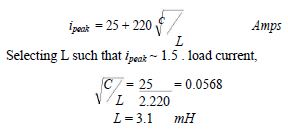Assuming that the capacitor charges to 70% of its original charge because of losses in the C- SCRM -L-D network, and it charges linearly when SCRA is again triggered,

q =1540 / 25 =61.6 μsec s

The SCR can therefore be successfully commutated.

The maximum current that can be commutated with the given Capacitor at the 220 V supply voltage is

For the 25 Amps load current the capacitor just enough would have a rating of

C = Iload .tq /(0.7.220) = (25.12) /154 =1.95 ≈ 2.0 μF

If the supply voltage is reduced by a factor K, the required capacitor rating increases by the same factor K for the same load current.

Class E – External pulse source for commutation

The transformer is designed with sufficient iron and air gap so as not to saturate. It is capable of carrying the load current with a small voltage drop compared with the supply voltage.
When SCR1 is triggered, current flows through the load and pulse transformer. To turn SCR1 off a positive pulse is applied to the cathode of the SCR from an external pulse generator via the pulse transformer. The capacitor C is only charged to about 1 volt and for the duration of the turn-off pulse it can be considered to have zero impedance. Thus the pulse from the transformer reverses the voltage across the SCR, and it supplies the reverse recovery current and holds the voltage negative for the required turn-off time.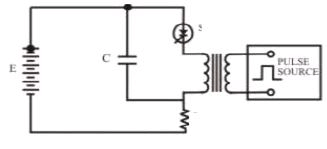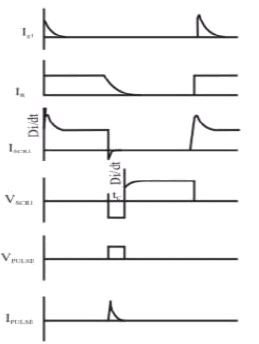Fig. 3.6 Class E, External pulse commutation

Class F, AC line commutated

If the supply is an alternating voltage, load current will flow during the positive half cycle. With a highly inductive load, the current may remain continuous for some time till the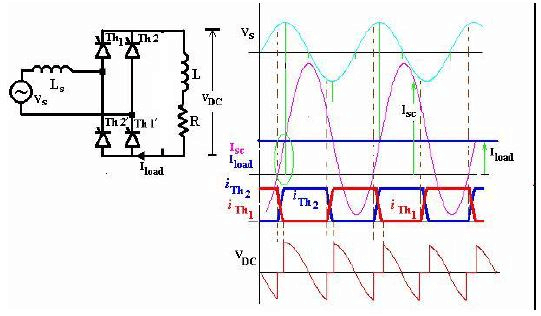Fig. 3.7 Class F, natural commutation by supply voltage

energy trapped in the load inductance is dissipated. During the negative half cycle, therefore, the SCR will turn off when the load current becomes zero 'naturally'. The negative polarity of the voltage appearing across the outgoing SCR turns it off if the voltage persists for the rated turn-off period of the device. The duration of the half cycle must be definitely longer than the turn-off time of the SCR.

The rectifier in Fig.3.6 is supplied from an single phase AC supply. The commutation process involved here is representative of that in a three phase converter. The converter has an input inductance Ls arising manly out of the leakage reactance of the supply transformer. Initially, SCRs Th1 and Th1' are considered to be conducting. The triggering angle for the converter is around 600. The converter is operating in the continuous conduction mode aided by the highly-inductive load.

When the incoming SCRs, Th2 and Th2' are triggered, the current through the incoming devices cannot rise instantaneously to the load current level. A circulating current Isc builds up in the short-circuited path including the supply voltage, Vs-Ls-Th1'- Th2 and Vs- Ls-Th2'-Th1 paths. This current can be described by: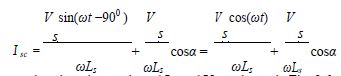where α the triggering angle and Isc and Vs as shown in Fig. 3.6.

This expression is obtained with the simplifying assumption that the input inductance contains no resistances. When the current rises in the incoming SCRs, which in the outgoing ones fall such that the total current remains constant at the load current level. When the current in the incoming ones reach load current level, the turn-off process of the outgoing ones is initiated. The reverse biasing voltage of these SCRs must continue till they reach their forward blocking state. As is evident from the above expression, the overlap period is a function of the triggering angle. It is lowest when α ~ 900. These SCRs being 'Converter grade', they have a larger turn-off time requirement of about 30-50 μsecs.

The period when both the devices conduct is known as the 'overlap period'. Since all SCRs are in conduction, the output voltage for this period is zero. If the 'fully-controlled' converter in Fig. 3.7 is used as an inverter with triggering angles > 900, the converter triggering can be delayed till the 'margin angle' which includes the overlap angle and the turn-off time of the SCR - both dependent on the supply voltages.

Rate of rise of forward voltage, dv/dt

The junctions of any semiconductor exhibit some unavoidable capacitance. A changing voltage impressed on this junction capacitance results in a current, I = C dv/dt. If this current is sufficiently large a regenerative action may occur causing the SCR to switch to the on state. This regenerative action is similar to that which occurs when gate current is injected. The critical rate of rise of off-state voltage is defined as the maximum value of rate of rise of forward voltage which may cause switching from the off-state to the on-state.

Since dv/dt turn-on is non-destructive, this phenomenon creates no problem in applications in which occasional false turn -on does not result in a harmful affect at the load. Heater application is one such case. However, at large currents where dv/dt turn-on is accompanied by partial turn-on of the device area a high di/dt occurs which then may be destructive.

The majority of inverter applications, however, would result in circuit malfunction due to dv/dt turn-on. One solution to this problem is to reduce the dv/dt imposed by the circuit to a value less than the critical dv/dt of the SCR being used. This is accomplished by the use of a circuit similar to those in Figure 3.8 to suppress excessive rate of rise of anode voltage. Z represents load impedance and circuit impedance. Variations of the basic circuit is also shown where the section of the network shown replaces the SCR and the R-C basic snubber.

Since circuit impedances are not usually well defined for a particular application, the values of R and C are often determined by experimental optimization. A technique can be used to simplify snubber circuit design by the use of nomographs which enable the circuit designer to select an optimized R-C snubber for a particular set of circuit operating conditions.

Another solution to the dv/dt turn-on problem is to use an SCR with higher dv/dt turn-on problem is to use an SCR with higher dv/dt capability. This can be done by selecting an SCR designed specially for high dv/dt applications, as indicated by the specification sheet. Emitter shorting is a manufacturing technique used to accomplish high dv/dt capability.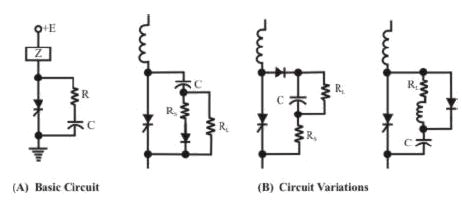Fig. 3.8 dv/dt supression circuits

Questions

• 1 For a Class D turn-off SCR, the load consists of a resistance only. If the supply voltage and SCR turn-off ratings are as in Problem # 1 calculate the required value of the commutating capacitor.

Ans: (Hints): The capacitor would now charge in an exponential manner. The time it takes to discharge from its reverse charged state once SCRA is triggered is the circuit turn-off time which must be in excess of the rated 12 μsecs.

• 2 For a Class F converter, will the overlap period rise with the leakage inductance of the converter? What happens to the output voltage?

Ans: Yes. The overlap time is directly related to the commutating inductance. The output voltage decreases. In fact, this inductor limits the maximum output current of the converter. The input current maximum would be as for a shorted network with the leakage inductance only present.

• 3 Can the output DC voltage be controlled in the above circuits?

Ans: Yes. Most of the above circuits are also called 'forced commutated' DC-DC chopper circuits.

The document Firing & Commutation Circuits of SCR | Power Electronics - Electrical Engineering (EE) is a part of the Electrical Engineering (EE) Course Power Electronics.
All you need of Electrical Engineering (EE) at this link: Electrical Engineering (EE)

## Power Electronics

5 videos|39 docs|66 tests

## FAQs on Firing & Commutation Circuits of SCR - Power Electronics - Electrical Engineering (EE)

 1. What is the purpose of firing circuits in SCR?Ans. The firing circuit in an SCR is responsible for providing the necessary gate current to trigger the SCR into conduction. It ensures that the SCR turns on at the desired moment and controls the power delivered to the load.
 2. How does a firing circuit work in SCR?Ans. A firing circuit generates a gate current pulse that is applied to the gate terminal of the SCR, triggering it into conduction. This pulse is typically generated by a control signal or a specific triggering mechanism, ensuring precise control over the SCR's conduction timing.
 3. What are the different types of firing circuits used in SCR?Ans. There are several types of firing circuits used in SCR applications. Some common types include resistance-capacitance (RC) firing circuits, pulse transformer firing circuits, and phase-controlled firing circuits. Each type offers different advantages and is used based on the specific application requirements.
 4. What is the role of commutation circuits in SCR?Ans. The commutation circuit in an SCR is responsible for turning off the SCR after it has been triggered into conduction. It ensures that the SCR stops conducting when the control signal or triggering mechanism is no longer present, preventing any damage or malfunction.
 5. What are the different methods used in commutation circuits for SCR?Ans. There are several methods used in commutation circuits for SCR, including natural commutation, forced commutation, and external commutation. Natural commutation relies on the load current dropping below the SCR's holding current to turn it off. Forced commutation uses additional circuitry to force the SCR to turn off, while external commutation involves using external devices to turn off the SCR. The choice of method depends on the specific application requirements.

## Power Electronics

5 videos|39 docs|66 testsExplore Courses for Electrical Engineering (EE) examSignup to see your scores go up within 7 days! Learn & Practice with 1000+ FREE Notes, Videos & Tests.
10M+ students study on EduRev
Track your progress, build streaks, highlight & save important lessons and more!
Related Searches

,

,

,

,

,

,

,

,

,

,

,

,

,

,

,

,

,

,

,

,

,

;